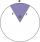# Triangle + system of equations - examples

1. Right triangle AlefThe obvod of a right triangle is 84 cm, the hypotenuse is 37 cm long. Determine the lengths of the legs.
2. Triangle ABCCalculate the sides of triangle ABC with area 1404 cm2 and if a: b: c = 12:7:18
3. Right triangleLegs of right are in ratio a:b = 6:8. Hypotenuse has a length of 61 cm. Calculate the perimeter and area of the triangle.
4. R triangleCalculate the area of a right triangle whose longer leg is 6 dm shorter than the hypotenuse and 3 dm longer than the shorter leg.
5. MediansCalculate the sides of a right triangle if the length of the medians to the legs are ta = 21 cm and tb=12 cm.
6. RT - hypotenuse and altitudeRight triangle BTG has hypotenuse g=117 m and altitude to g is 54 m. How long are hypotenuse segments?
7. Triangle anglesThe angles α, β, γ in triangle ABC are in the ratio 6:2:6. Calculate size of angles.
8. Circular sectorI have a circular sector with a length 15 cm with an unknown central angle. It is inscribed by a circle with radius 5 cm. What is the central angle alpha in the circular sector?
9. Trapezoidtrapezoid ABCD a = 35 m, b=28 m c = 11 m and d = 14 m. How to calculate its area?
10. Angles in a triangleThe angles of the triangle ABC make an arithmetic sequence with the largest angle γ=83°. What sizes have other angles in a triangle?
11. Angles in triangleThe triangle is ratio of the angles β:γ = 6:8. Angle α is 40° greater than β. What are the size of angles of the triangle?
12. Isosceles triangleThe perimeter of an isosceles triangle is 112 cm. The length of the arm to the length of the base is at ratio 5:6. Find the triangle area.
13. Angles of the triangleABC is a triangle. The size of the angles alpha, beta are in a ratio 4: 7. The angle gamma is greater than the angle alpha by a quarter of a straight angle. Determine angles of the triangle ABC.
14. Angles ratioThe internal angles of a triangle are in ratio 1:4:5 What kind of triangle is it? (solve internal angles and write down and discuss)
15. CloudsFrom two points A and B on the horizontal plane was observed forehead cloud above the two points under elevation angle 73°20' and 64°40'. Points A , B are separated by 2830 m. How high is the cloud?
16. Angle in RTDetermine the size of the smallest internal angle of a right triangle whose sides constitutes sizes consecutive members of arithmetic progressions.
17. Diagonal 20Diagonal pathway for the rectangular town plaza whose length is 20 m longer than the width. if the pathway is 20 m shorter than twice the width. How long should the pathway be?
18. GarageThere are two laths in the garage opposite one another: one 2 meters long and the second 3 meters long. They fall against each other and stay against the opposite walls of the garage and both laths cross 70 cm above the garage floor. How wide is the garag
19. Is right triangleOne angle of the triangle is 36° and the remaining two are in the ratio 3:5. Determine whether triangle is a rectangular triangle.
20. Angles of a triangleIn the triangle ABC, the angle beta is 15° greater than the angle alpha. The remaining angle is 30° greater than the sum of the angles alpha and beta. Calculate the angles of a triangle.

Do you have an interesting mathematical example that you can't solve it? Enter it, and we can try to solve it.

To this e-mail address, we will reply solution; solved examples are also published here. Please enter e-mail correctly and check whether you don't have a full mailbox.

Do you have a system of equations and looking for calculator system of linear equations? See also our trigonometric triangle calculator. See also more information on Wikipedia.